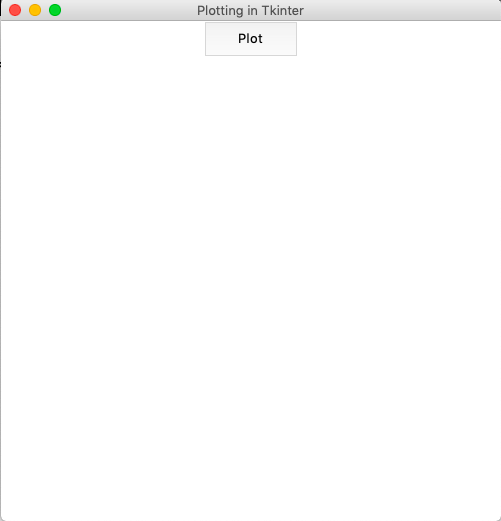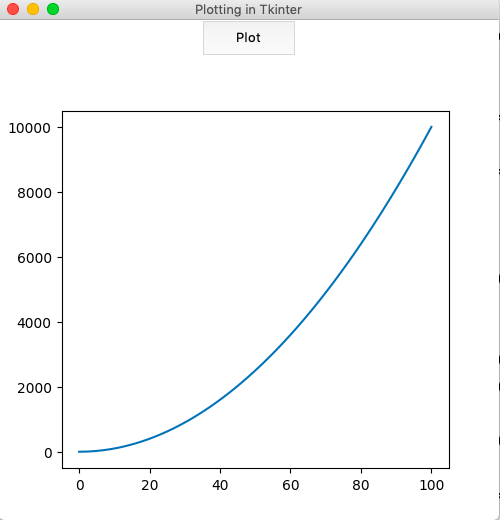Related Articles
How to embed Matplotlib charts in Tkinter GUI?
• Last Updated : 10 Jul, 2020

Prerequisite: Introduction to Tkinter | Introduction to Matplotlib

When Matplotlib is used from Python shell, the plots are displayed in a default window. The plots can be embedded in many graphical user interfaces like wxpython, pygtk, or Tkinter. These various options available as a target for the output plot are referred to as ‘backends‘. There are various modules available in matplotlib.backend for choosing the backend. One such module is backend_tkagg which is useful for embedding plots in Tkinter.

#### Creating the Tkinter Application :

First, let us create a basic Tkinter application with the main window and one button which can be used to display the plot.

## Python3

 `# import all classes/methods``# from the tkinter module``from` `tkinter ``import` `*`` ` `# The main tkinter window``window ``=` `Tk()`` ` `# setting the title and ``window.title(``'Plotting in Tkinter'``)`` ` `# setting the dimensions of ``# the main window``window.geometry(``"500x500"``)`` ` `# button that would displays the plot``plot_button ``=` `Button(master ``=` `window,``                     ``height ``=` `2``,``                     ``width ``=` `10``,``                    ``text ``=` `"Plot"``)``# place the button``# into the window``plot_button.pack()`` ` `# run the gui``window.mainloop()`

Output :#### Embedding the Plot:

First, we need to create the figure object using the Figure() class. Then, a Tkinter canvas(containing the figure) is created using FigureCanvasTkAgg() class. Matplotlib charts by default have a toolbar at the bottom. When working with Tkinter, however, this toolbar needs to be embedded in the canvas separately using the NavigationToolbar2Tk() class.
In the implementation below, a simple graph for:is plotted. The plot function is bound to a button that displays the figure when pressed.

## Python3

 `from` `tkinter ``import` `*` `from` `matplotlib.figure ``import` `Figure``from` `matplotlib.backends.backend_tkagg ``import` `(FigureCanvasTkAgg, ``NavigationToolbar2Tk)`` ` `# plot function is created for ``# plotting the graph in ``# tkinter window``def` `plot():`` ` `    ``# the figure that will contain the plot``    ``fig ``=` `Figure(figsize ``=` `(``5``, ``5``),``                 ``dpi ``=` `100``)`` ` `    ``# list of squares``    ``y ``=` `[i``*``*``2` `for` `i ``in` `range``(``101``)]`` ` `    ``# adding the subplot``    ``plot1 ``=` `fig.add_subplot(``111``)`` ` `    ``# plotting the graph``    ``plot1.plot(y)`` ` `    ``# creating the Tkinter canvas``    ``# containing the Matplotlib figure``    ``canvas ``=` `FigureCanvasTkAgg(fig,``                               ``master ``=` `window)  ``    ``canvas.draw()`` ` `    ``# placing the canvas on the Tkinter window``    ``canvas.get_tk_widget().pack()`` ` `    ``# creating the Matplotlib toolbar``    ``toolbar ``=` `NavigationToolbar2Tk(canvas,``                                   ``window)``    ``toolbar.update()`` ` `    ``# placing the toolbar on the Tkinter window``    ``canvas.get_tk_widget().pack()`` ` `# the main Tkinter window``window ``=` `Tk()`` ` `# setting the title ``window.title(``'Plotting in Tkinter'``)`` ` `# dimensions of the main window``window.geometry(``"500x500"``)`` ` `# button that displays the plot``plot_button ``=` `Button(master ``=` `window, ``                     ``command ``=` `plot,``                     ``height ``=` `2``, ``                     ``width ``=` `10``,``                     ``text ``=` `"Plot"``)`` ` `# place the button ``# in main window``plot_button.pack()`` ` `# run the gui``window.mainloop()`

Output :Attention geek! Strengthen your foundations with the Python Programming Foundation Course and learn the basics.

To begin with, your interview preparations Enhance your Data Structures concepts with the Python DS Course.

My Personal Notes arrow_drop_up